# How to analyze log data with Python and Apache Spark

## Case study with NASA logs to show how Spark can be leveraged for analyzing data at scale.Image by :
Opensource.com

## Subscribe now

Get the highlights in your inbox every week.

In part one of this series, we began by using Python and Apache Spark to process and wrangle our example web logs into a format fit for analysis, a vital technique considering the massive amount of log data generated by most organizations today. We set up environment variables, dependencies, loaded the necessary libraries for working with both DataFrames and regular expressions, and of course loaded the example log data. Then we wrangled our log data into a clean, structure, and meaningful format. Here in part two, we focus on analyzing that data.

## Data analysis on our web logs

Now that we have a DataFrame containing the parsed and cleaned log file as a data frame, we can perform some interesting exploratory data analysis (EDA) to try and get some interesting insights!

### Content size statistics

Let’s compute some statistics regarding the size of content our web server returns. In particular, we’d like to know the average, minimum, and maximum content sizes.

We compute these statistics by calling `.describe()` on the `content_size` column of `logs_df`. The `.describe()` function returns the `count`, `mean`, `stddev`, `min`, and `max `of a given column in this format:

```content_size_summary_df = logs_df.describe(['content_size'])
content_size_summary_df.toPandas()```

## visualizing_and_analyzing_nasa_logs_figure_1.png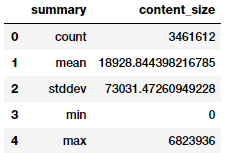Stastical analysis regarding the size of content your web server returns.

Alternatively, we can use SQL to directly calculate these statistics. The `pyspark.sql.functions` module has many useful functions, see the documentation to learn more.

After we apply the `.agg()` function, we call `toPandas()` to extract and convert the result into a pandas DataFrame, which offers better formatting on Jupyter Notebook:

```from pyspark.sql import functions as F

(logs_df.agg(F.min(logs_df['content_size']).alias('min_content_size'),
F.max(logs_df['content_size']).alias('max_content_size'),
F.mean(logs_df['content_size']).alias('mean_content_size'),
F.stddev(logs_df['content_size']).alias('std_content_size'),
F.count(logs_df['content_size']).alias('count_content_size'))
.toPandas())```

## visualizing_and_analyzing_nasa_logs_figure_2_600.png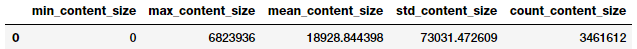The same data reformatted into a pandas dataframe.

When we validate the results, we see that they are the same as expected.

### HTTP status code analysis

Next, let’s look at the log's status code values to see which status code values appear and how many times. We again start with `logs_df`, group by the `status` column, apply the `.count()` aggregation function, and then sort by the `status` column:

```status_freq_df = (logs_df
.groupBy('status')
.count()
.sort('status')
.cache())
print('Total distinct HTTP Status Codes:', status_freq_df.count())                     ```

`Total Distinct HTTP Status Codes: 8`

Let’s take a look at each status code's occurrences in the form of a frequency table:

```status_freq_pd_df = (status_freq_df
.toPandas()
.sort_values(by=['count'],
ascending=False))
status_freq_pd_df```

## visualizing_and_analyzing_nasa_logs_figure_3.png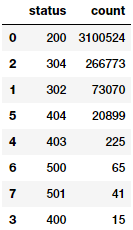How many times each status code appears in your log.

Looks like the most frequent status code is 200—OK—which is a good sign that things are working normally most of the time. Let’s visualize this:

```import matplotlib.pyplot as plt
import seaborn as sns
import numpy as np
%matplotlib inline

sns.catplot(x='status', y='count', data=status_freq_pd_df,
kind='bar', order=status_freq_pd_df['status'])```

## visualizing_and_analyzing_nasa_logs_figure_4.png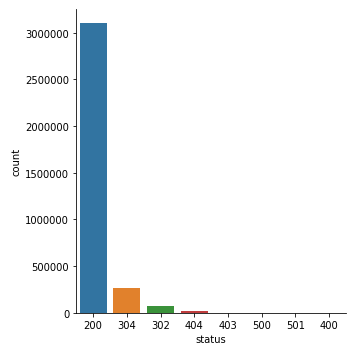HTTP status code occurrences in a bar chart.

Not too bad. But several status codes are nearly invisible due to the data's huge skew. Let’s do a log transform and see if things improve. Usually log transforms help us transform highly skewed data to an approximate normal distribution, so that we can visualize the data distribution in a more comprehensible manner:

```log_freq_df = status_freq_df.withColumn('log(count)',
F.log(status_freq_df['count']))
log_freq_df.show()```

## visualizing_and_analyzing_nasa_logs_figure_5.png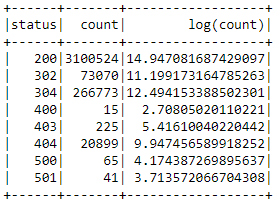Error code frequency as a log transform.

The results definitely look good and seem to have handled the skew, let’s verify this by visualizing this data:

```log_freq_pd_df = (log_freq_df
.toPandas()
.sort_values(by=['log(count)'],
ascending=False))
sns.catplot(x='status', y='log(count)', data=log_freq_pd_df,
kind='bar', order=status_freq_pd_df['status'])```

## visualizing_and_analyzing_nasa_logs_figure_6.png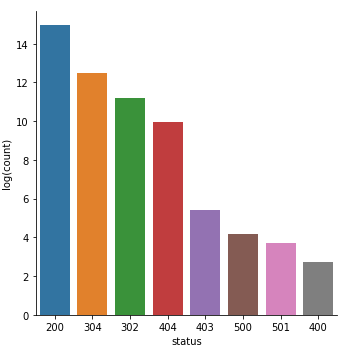HTTP status code frequency bar chart, after a log transform.

This chart definitely looks much better and less skewed, giving us a better idea of the distribution of status codes!

### Analyzing frequent hosts

Let’s look at hosts that access the server frequently by getting the total count of accesses by each host, sorting by the number of accesses, and displaying only the top 10 most frequent hosts:

```host_sum_df =(logs_df
.groupBy('host')
.count()
.sort('count', ascending=False).limit(10))

host_sum_df.show(truncate=False)```

## visualizing_and_analyzing_nasa_logs_figure_7.png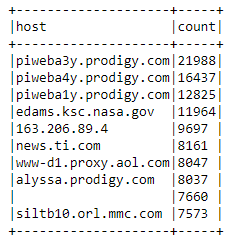Hosts that frequently access the server sorted by number of accesses.

This table looks good, but let’s inspect the blank record in row number 9 more closely:

```host_sum_pd_df = host_sum_df.toPandas()
host_sum_pd_df.iloc[‘host’]```

`''`

Looks like one of the top hostnames is an empty string. This example teaches us a valuable lesson: don't just check for nulls when data wrangling, also check for empty strings.

### Display the top 20 most frequent endpoints

Now, let’s visualize the number of endpoint URI hits in the log. To perform this task, start with logs_df and then group by the endpoint column, aggregate by count, and sort in descending order like the previous example:

```paths_df = (logs_df
.groupBy('endpoint')
.count()
.sort('count', ascending=False).limit(20))

paths_pd_df = paths_df.toPandas()
paths_pd_df            ```

## visualizing_and_analyzing_nasa_logs_figure_8.png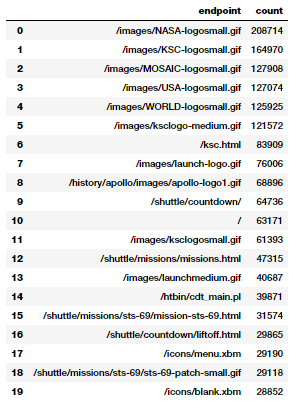A table showing the number of hits to each endpoint URI in descending order.

Not surprisingly, the most accessed assets are GIFs, the home page, and some CGI scripts.

### Display the top 10 error endpoints

What are the top 10 endpoints requested which did not have return code 200 (HTTP Status OK)? To find out, we create a sorted list containing the endpoints and the number of times they were accessed with a non-200 return code, and then show the top 10:

```not200_df = (logs_df
.filter(logs_df['status'] != 200))

error_endpoints_freq_df = (not200_df
.groupBy('endpoint')
.count()
.sort('count', ascending=False)
.limit(10)
)

error_endpoints_freq_df.show(truncate=False)                          ```

## visualizing_and_analyzing_nasa_logs_figure_9.pngA table displaying the top ten error endpoints and their frequency.

Looks like GIFs (animated/static images) are failing to load the most. Why would that be? Well given that these logs are from 1995, and given the internet speed we had back then, I’m not surprised!

### Total number of unique hosts

How many unique hosts visited the NASA website in these two months? We can find this out with a few transformations:

```unique_host_count = (logs_df
.select('host')
.distinct()
.count())
unique_host_count```

`137933`

### Number of unique daily hosts

For an advanced example, let’s look at how to determine the number of unique hosts on a day-by-day basis. Here we’d like a DataFrame that includes the day of the month and the associated number of unique hosts for that day, sorted by increasing day of the month.

Think about the steps you need to perform for this task. Since each of these logs only covers a single month, you can ignore the month issue, at least. For data spanning multiple months, we would need to consider both month and day when doing the necessary aggregations. You may want to use the `pyspark.sql.functions` module's `dayofmonth()` function (which we have already imported as `F `at the beginning of this tutorial`)`.

Start with `host_day_df`, which is a DataFrame with two columns:

## visualizing_and_analyzing_nasa_logs_figure_10.png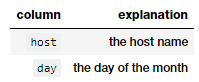The columns in the host_day_df dataframe.

There is one row in this DataFrame for each row in `logs_df`. Essentially, we're just transforming each row. For example, for this row:

``unicomp6.unicomp.net - - [01/Aug/1995:00:35:41 -0400] "GET /shuttle/missions/sts-73/news HTTP/1.0" 302 -``

your` host_day_df` should have `unicomp6.unicomp.net 1`

```host_day_df = logs_df.select(logs_df.host,
F.dayofmonth('time').alias('day'))
host_day_df.show(5, truncate=False)```

## visualizing_and_analyzing_nasa_logs_figure_11.png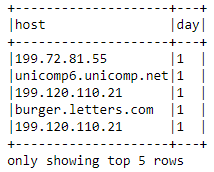The top five hosts making requests on the first day.

Next is `host_day_distinct_df`, which is a DataFrame with the same columns as `host_day_df`, but with duplicate `(day, host)` rows removed:

```host_day_df = logs_df.select(logs_df.host,
F.dayofmonth('time').alias('day'))
host_day_df.show(5, truncate=False)```

## visualizing_and_analyzing_nasa_logs_figure_12.pnghost_day_distinct_df gives the same output as host_day_df, but with duplicate rows removed.

Another option is `daily_unique_hosts_df`, which is a DataFrame with two columns, one different from the previous DataFrames:

## visualizing_and_analyzing_nasa_logs_figure_14.png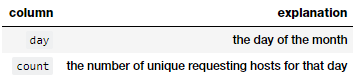Columns shown by daily_unique_hosts_df.

```def_mr = pd.get_option('max_rows')
pd.set_option('max_rows', 10)

daily_hosts_df = (host_day_distinct_df
.groupBy('day')
.count()
.sort("day"))

daily_hosts_df = daily_hosts_df.toPandas()
daily_hosts_df```

## visualizing_and_analyzing_nasa_logs_figure_13.png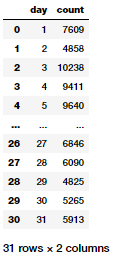daily_unique_hosts_df shows the day of the month and the number of unique hosts making requests that day.

This result gives us a nice DataFrame showing the total number of unique hosts per day. Let’s visualize this:

```c = sns.catplot(x='day', y='count',
data=daily_hosts_df,
kind='point', height=5,
aspect=1.5)```

## visualizing_and_analyzing_nasa_logs_figure_15.png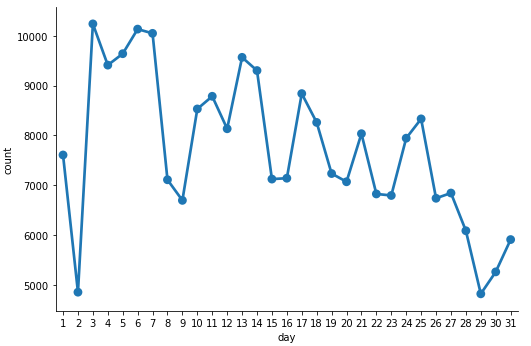Unique hosts per day charted daily_unique_hosts_df.

### Average number of daily requests per host

In the previous example, we looked at one way to determine the number of unique hosts in the entire log on a day-by-day basis. Let’s now find the average number of requests made per host to the NASA website per day. Here, we’d like a DataFrame sorted by increasing day of the month which includes the day of the month and the associated number of average requests made for that day per host:

```daily_hosts_df = (host_day_distinct_df
.groupBy('day')
.count()
.select(col("day"),
col("count").alias("total_hosts")))

total_daily_reqests_df = (logs_df
.select(F.dayofmonth("time")
.alias("day"))
.groupBy("day")
.count()
.select(col("day"),
col("count").alias("total_reqs")))

avg_daily_reqests_per_host_df = total_daily_reqests_df.join(daily_hosts_df, 'day')
avg_daily_reqests_per_host_df = (avg_daily_reqests_per_host_df
.withColumn('avg_reqs', col('total_reqs') / col('total_hosts'))
.sort("day"))
avg_daily_reqests_per_host_df = avg_daily_reqests_per_host_df.toPandas()
avg_daily_reqests_per_host_df```

## visualizing_and_analyzing_nasa_logs_figure_16.png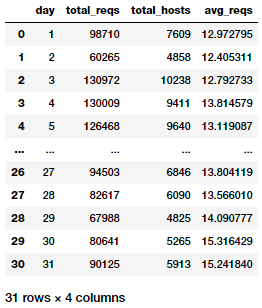The average number of daily requests per host via avg_daily_reqests_per_host_df.

Now we can visualize the average daily requests per host:

```c = sns.catplot(x='day', y='avg_reqs',
data=avg_daily_reqests_per_host_df,
kind='point', height=5, aspect=1.5)```

## visualizing_and_analyzing_nasa_logs_figure_17.png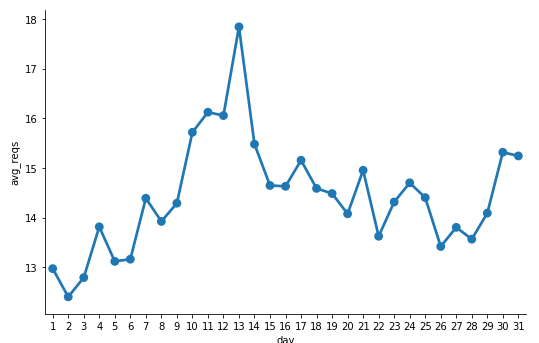The average number of daily requests per host charted.

It looks like day 13 got the maximum number of requests per host.

### Counting 404 response codes

Create a DataFrame containing only log records with a 404 status code (Not Found). We make sure to `cache()` the `not_found_df` DataFrame as we will use it in the rest of the examples here. How many 404 records do you think are in the logs?

```not_found_df = logs_df.filter(logs_df["status"] == 404).cache()
print(('Total 404 responses: {}').format(not_found_df.count()))```

`Total 404 responses: 20899`

### Listing the top twenty 404 response code endpoints

Using the DataFrame that we cached earlier—containing only log records with a 404 response code—we will now print out a list of the top twenty endpoints generating the most 404 errors.   Remember, whenever you generate top endpoints, they should be in sorted order:

```endpoints_404_count_df = (not_found_df
.groupBy("endpoint")
.count()
.sort("count", ascending=False)
.limit(20))

endpoints_404_count_df.show(truncate=False)```

## visualizing_and_analyzing_nasa_logs_figure_18.png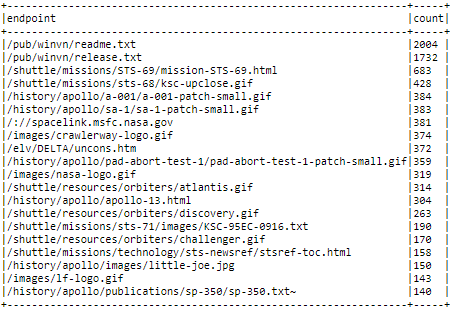The top 20 response code endpoints, sorted, thanks to endpoints_404_count_df

### Listing the top twenty 404 response code hosts

Using the DataFrame that we cached earlier which contains only log records with a 404 response code, we can now print out a list of the top twenty hosts that generate the most 404 errors.  Again, remember that top hosts should be in sorted order:

```hosts_404_count_df = (not_found_df
.groupBy("host")
.count()
.sort("count", ascending=False)
.limit(20))

hosts_404_count_df.show(truncate=False)```

## visualizing_and_analyzing_nasa_logs_figure_19.png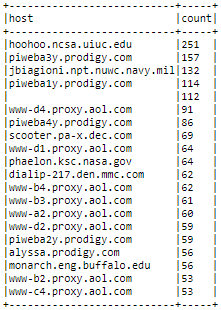The top twenty 404 response code hosts via hosts_404_count_df.

This output gives us a good idea which hosts end up generating the most 404 errors for the NASA webpage.

### Visualizing 404 errors per day

Let’s now explore our 404 records temporally (by time). Similar to the example showing the number of unique daily hosts, we'll break down the 404 requests by day and sor the daily counts by day in `errors_by_date_sorted_df`:

```errors_by_date_sorted_df = (not_found_df
.groupBy(F.dayofmonth('time').alias('day'))
.count()
.sort("day"))

errors_by_date_sorted_pd_df = errors_by_date_sorted_df.toPandas()
errors_by_date_sorted_pd_df```

## visualizing_and_analyzing_nasa_logs_figure_20.png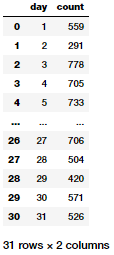404 errors per day via errors_by_date_sorted_df.

Now let’s visualize the total 404 errors per day now:

```c = sns.catplot(x='day', y='count',
data=errors_by_date_sorted_pd_df,
kind='point', height=5, aspect=1.5)```

## visualizing_and_analyzing_nasa_logs_figure_21.png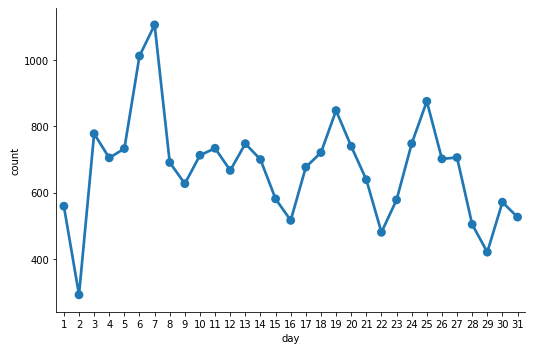Total 404 errors per day.

### Top three days for 404 errors

Based on the earlier plot, what are the top three days of the month with the most 404 errors? Knowing this can help us diagnose and drill down further into these specfic days to figure out what might have gone wrong (server issues, DNS issues, denial of service, latency problems, maintenance and so on). We can leverage our previously created errors_by_date_sorted_df DataFrame to answer this question:

```(errors_by_date_sorted_df
.sort("count", ascending=False)
.show(3))```

## visualizing_and_analyzing_nasa_logs_figure_22.png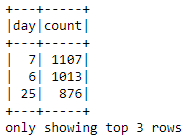The top 3 days of 404 errors via errors_by_date_sorted_df.

### Visualizing hourly 404 errors

Using the DataFrame not_found_df that we cached earlier, we can now group and sort by hour of the day in increasing order. We'll use this process to create a DataFrame containing the total number of 404 responses for HTTP requests for each hour of the day (midnight starts at 0). Then we will build a visualization from the DataFrame.

```hourly_avg_errors_sorted_df = (not_found_df
.groupBy(F.hour('time')
.alias('hour'))
.count()
.sort('hour'))
hourly_avg_errors_sorted_pd_df = hourly_avg_errors_sorted_df.toPandas()

c = sns.catplot(x='hour', y='count',
data=hourly_avg_errors_sorted_pd_df,
kind='bar', height=5, aspect=1.5)```

## visualizing_and_analyzing_nasa_logs_figure_23.png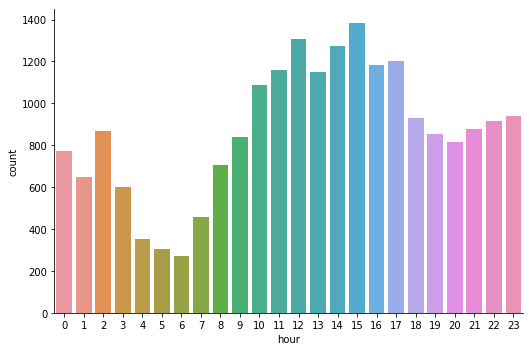Total 404 errors per hour in a bar chart.

Looks like total 404 errors occur the most in the afternoon and the least in the early morning. We can now reset the maximum rows displayed by pandas to the default value since we had changed it earlier to display a limited number of rows.

``pd.set_option(‘max_rows’, def_mr)``

## Conclusion

We took a hands-on approach to data wrangling, parsing, analysis and visualization at scale on a very common yet essential case-study on Log Analytics. While the data we worked on here may not be ‘Big Data’ from a size or volume perspective, these techniques and methodologies are generic enough to scale for larger volumes of data. I hope this exercise gave you ideas on how you might leverage open source frameworks like Apache Spark to work with your own structured and semi-structured data!

You can find all the code and analyses accompanying this article in my GitHub repository. Also, you can find a step-by-step approach in this Jupyter Notebook.

Interested in understanding Spark SQL and DataFrames? Check out my hands-on tutorial on opensource.com!